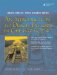# Subobjects

An object can contain another object, in which case the contained object is considered to be a subobject. In Example 2.9, each Square object has two Point subobjects. Notice that Point has a function named ~Point. We discuss this kind of function in the next section.

Example 2.9. src/subobject/subobject.h

 ```[ . . . . ] class Point { public: Point(int xx, int yy) : m_x(xx), m_y(yy){} ~Point() { cout << "point destroyed: (" << m_x << "," << m_y << ")" << endl; } private: int m_x, m_y; }; class Square { public: Square(int ulx, int uly, int lrx, int lry) : m_UpperLeft(ulx, uly), m_LowerRight (lrx, lry) <-- 1 {} Square(const Point& ul, const Point& lr) : m_UpperLeft(ul), m_LowerRight(lr) {} <-- 2 private: Point m_UpperLeft, m_LowerRight; <-- 3 }; [ . . . . ] ```   (1)Initialization is required because there is no default ctor. (2)Initialize using the implicitly generated Point copy ctor. (3)embedded subobjects

Whenever an instance of Square is created, each of its subobjects is created with it, so that the three objects occupy contiguous chunks of memory.

The Square is composed of two Point objects.

Because Point has no default constructor, we must properly initialize for each Point subobject in the member initialization list of Square (see Example 2.10).

Example 2.10. src/subobject/subobject.cpp

 ```#include "subobject.h" int main() { Square sq1(3,4,5,6); Point p1(2,3), p2(8, 9); Square sq2(p1, p2); } ```

Even though no destructor was defined for Square, each of its Point subobjects is properly destroyed whenever the containing object is. This is an example of composition.

```point destroyed: (8,9)
point destroyed: (2,3)
point destroyed: (8,9)
point destroyed: (2,3)
point destroyed: (5,6)
point destroyed: (3,4)
```An Introduction to Design Patterns in C++ with Qt 4
ISBN: 0131879057
EAN: 2147483647
Year: 2004
Pages: 268